Logic

# Castling Puzzles

In the following position, what is the smallest number of moves White can make to force checkmate?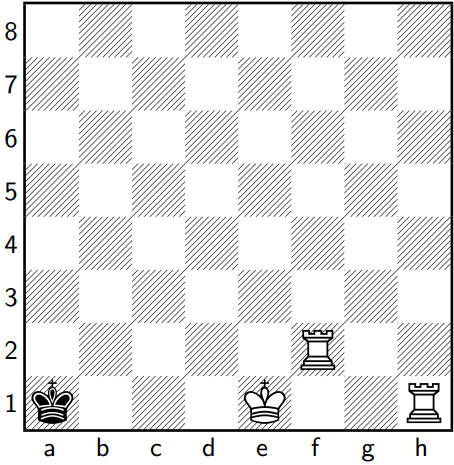(For this and all other problems in this quiz, if it appears castling may be possible then you may assume it is possible.)

In the following position, what is the smallest number of moves White can make to force checkmate?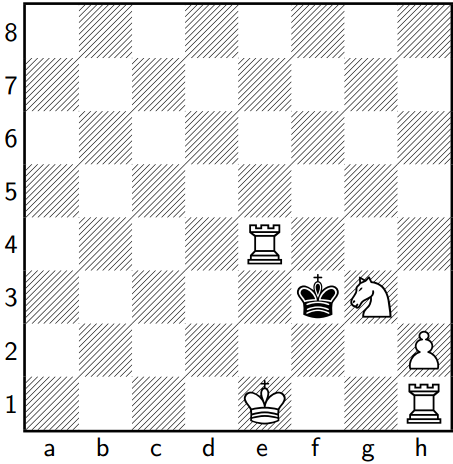In the given position, which of the following moves should White make to ensure a winning position?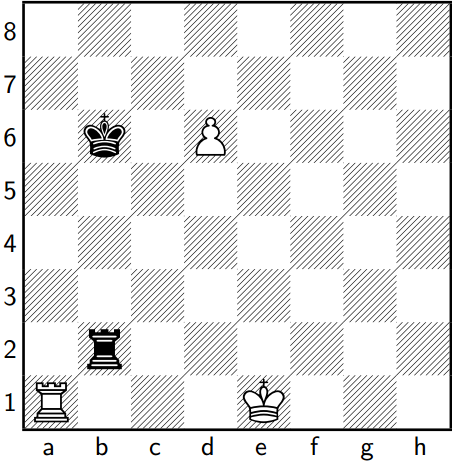In the following position, what is the smallest number of moves White can make to force checkmate?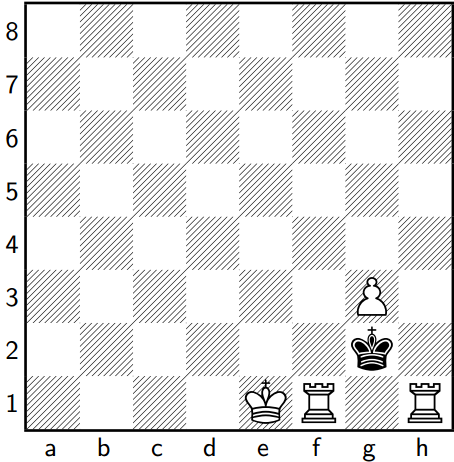In the following position, what is the smallest number of moves White can make to force checkmate?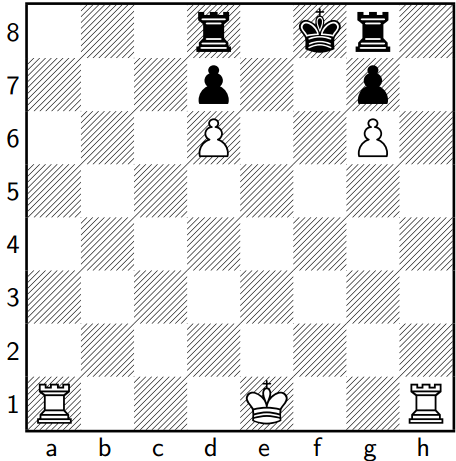×